## UGC NET CS 2016 July- paper-3

 Question 1
Which of the following is a sequential circuit?
 A Multiplexer B Decoder C Counter D Full adder
Digital-Logic-Design       Sequential-Circuits
Question 1 Explanation:
Combinational circuits have no memory. Combinational circuits are defined as the time independent circuits which do not depend upon previous inputs to generate any output are termed as combinational circuits.
Examples: Encoder, Decoder, Multiplexer, Demultiplexer
Sequential circuits have memory. Sequential circuits are those which are dependent on clock cycles and depends on present as well as past inputs to generate any output.
Example: Flip-flops, Counters
 Question 2
8085 microprocessor has _____ hardware interrupts.
 A 2 B 3 C 4 D 5
Computer-Organization       Microprocessor
Question 2 Explanation:Question 3
Which of the following in 8085 microprocessor performs HL = HL + DE ?
Computer-Organization       Microprocessor
Question 3 Explanation:
DAD: - Add register pair to HL register. The 16-bit contents of the specified register pair are added to the contents of the HL register and the sum is stored in the HL register. The contents of the source register pair are not altered. If the result is larger than 16 bits, the CY flag is set. No other flags are affected.
HL = HL + DE means DAD D
 Question 4
The register that stores all interrupt requests is:
 A Interrupt mask register B Interrupt service register C Interrupt request register D Status register
Computer-Organization       Interruption
Question 4 Explanation:
Status register(SR):
→ A status register is a collection of status flag bits for a processor.
→ The status register is a hardware register that contains information about the state of the processor. Individual bits are implicitly or explicitly read and/or written by the machine code instructions executing on the processor.
→ The status register lets an instruction take action contingent on the outcome of a previous instruction.
Interrupt service register(ISR):
→ Interrupt service routine(ISR) is a special block of code associated with a specific interrupt condition.
→ Interrupt handlers are initiated by hardware interrupts, software interrupt instructions, or software exceptions, and are used for implementing device drivers or transitions between protected modes of operation, such as system calls.
→ This register enables or masks interrupts from being triggered on the external pins of the Cache Controller.
→ The IMR is used to disable (Mask) or enable (Unmask) individual interrupt request inputs. This is also an 8-bit register.
Interrupt request register(IRR):
→ It stores all the interrupt inputs that are requesting service.
→ It is an 8-bit register, one bit for each interrupt request.
→ It keeps track of which interrupt inputs are asking for service. If an interrupt input is unmasked, and has an interrupt signal on it, then the corresponding bit in the IRR will be set.
→ The content of this register can be read to know the status of pending interrupts.
 Question 5
The _____ addressing mode is similar to register indirect addressing mode, except that an offset is added to the contents of the register. The offset and register are specified in the instruction.
 A Base indexed B Base indexed plus displacement C Indexed D Displacement
 Question 6
In _____ method, the word is written to the block in both the cache and main memory, in parallel.
 A Write through B Write back C Write protected D Direct mapping
Computer-Organization       Cache
Question 6 Explanation:
→ A cache memory contains copies of data stored in the main memory. When a change of data in a cache takes place (ex. a modification due to a processor write) the contents of the main memory and cache memory cells with the same address, are different. To eliminate this lack of data coherency two methods are applied:
write through, the new cache contents is written down to the main memory immediately after the write to the cache memory,
write back, the new cache contents is not written down to the main memory immediately after the change, but only when the given block of data is replaced by a new block fetched from the main memory or an upper level cache. After a data write to the cache, only state bits are changed in the modified block, indicating that the block has been modified (a dirty block).
The write back updating is more time efficient, since the block cells that were modified many times while being in the cache, are updated in the main memory only once.
 Question 7
Which of the following statements concerning Object-Oriented databases is FALSE?
 A Objects in an object-oriented database contain not only data but also methods for processing the data. B Object-oriented databases store computational instructions in the same place as the data. C Object-oriented databases are more adept at handling structured (analytical) data than relational databases. D Object-oriented databases store more types of data than relational databases and access that data faster.
Database-Management-System       OODBMS
Question 7 Explanation:
Object-oriented databases have adopted many of the concepts that were developed originally for object-oriented programming languages.
These include:
Object identity: Objects have unique identities that are independent of their attribute values and are generated by the ODMS.
Type constructors: Complex object structures can be constructed by applying in a nested manner a set of basic constructors, such as tuple, set, list, array, and bag.
Encapsulation of operations: Both the object structure and the operations that can be applied to individual objects are included in the type definitions.
Programming language compatibility: Both persistent and transient objects are handled seamlessly. Objects are made persistent by being reachable from a persistent collection (extent) or by explicit naming.
Type hierarchies and inheritance: Object types can be specified by using a type hierarchy, which allows the inheritance of both attributes and methods (operations) of previously defined types. Multiple inheritance is allowed in some models.
Extents: All persistent objects of a particular type can be stored in an extent. Extents corresponding to a type hierarchy have set/subset constraints enforced on their collections of persistent objects.
Polymorphism and operator overloading: Operations and method names can be overloaded to apply to different object types with different implementations.
 Question 8
In distributed databases, location transparency allows for database users, programmers and administrators to treat the data as if it is at one location. A SQL query with location transparency needs to specify:
 A Inheritances B Fragments C Locations D Local formats
Database-Management-System       Databases
Question 8 Explanation:
In distributed databases, location transparency allows for database users, programmers and administrators to treat the data as if it is at one location. A SQL query with location transparency needs to specify fragments.
 Question 9
Consider the relations R(A, B) and S(B, C) and the following four relational algebra queries over R and S:

I. ΠA, B (R ⨝ S)

II. R ⨝ ΠB(S)

III. R ∩ (ΠA(R) × ΠB(S))

IV. ΠA, R.B (R × S)

where R⋅B refers to the column B in table R.

One can determine that:

 A I, III and IV are the same query. B II, III and IV are the same query. C I, II and IV are the same query. D I, II and III are the same query.
Database-Management-System       Relational-Algebra
Question 9 Explanation: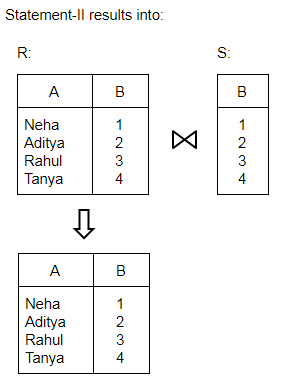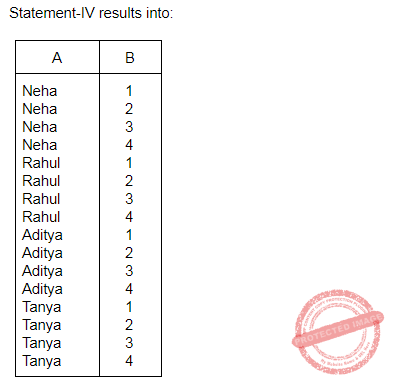Question 10
Which of the following statements is TRUE?

D1 : The decomposition of the schema R(A, B, C) into R1(A, B) and R2 (A, C) is always lossless.

D2 : The decomposition of the schema R(A, B, C, D, E) having AD → B, C → DE, B → AE and AE → C, into R1 (A, B, D) and R2 (A, C, D, E) is lossless.

 A Both D1 and D2 B Neither D1 nor D2 C Only D1 D Only D2
Database-Management-System       Functional-Dependency
Question 10 Explanation:
Statement(1) is incorrect because functional dependencies of relation R are not given, so we can't say whether the decomposition of R into R1 and R2 is lossless or not.Question 11
Consider the following ORACLE relations :

R (A, B, C) = {<1, 2, 3>, <1, 2, 0>, <1, 3, 1>, <6, 2, 3>, <1, 4, 2>, <3, 1, 4> }

S (B, C, D) = {<2, 3, 7>, <1, 4, 5>, <1, 2, 3>, <2, 3, 4>, <3, 1, 4>}.

Consider the following two SQL queries SQ1 and SQ2 :

SQ1 : SELECT R⋅B, AVG (S⋅B) FROM R, S WHERE R⋅A = S⋅C AND S⋅D < 7 GROUP BY R⋅B;

SQ2 : SELECT DISTINCT S⋅B, MIN (S⋅C) FROM S GROUP BY S⋅B HAVING COUNT (DISTINCT S⋅D) > 1;

If M is the number of tuples returned by SQ1 and N is the number of tuples returned by SQ2 then

 A M = 4, N = 2 B M = 5, N = 3 C M = 2, N = 2 D M = 3, N = 3
Database-Management-System       SQL
Question 11 Explanation:Question 12
Semi-join strategies are techniques for query processing in distributed database system. Which of the following is a semi-join technique ?
 A Only the joining attributes are sent from one site to another and then all of the rows are returned. B All of the attributes are sent from one site to another and then only the required rows are returned. C Only the joining attributes are sent from one site to another and then only the required rows are returned. D All of the attributes are sent from one site to another and then only the required rows are returned.
Database-Management-System       Relational-Algebra
Question 12 Explanation:
A semi-join returns one copy of each row in the first table for which at least one match is found. Semi-join is like EXCEPT command in SQL.
 Question 13
Consider the Breshenham’s circle generation algorithm for plotting a circle with centre (0, 0) and radius ‘r’ units in first quadrant. If the current point is (xi , yi ) and decision parameter is pi then what will be the next point (xi + 1, yi + 1 + 1) and updated decision parameter pi + 1 for pi ≥ 0?
 A xi + 1 = xi+ 1 yi + 1 = yi pi + 1 = pi + 4xi + 6 B xi + 1 = xi + 1 yi + 1 = yi - 1 pi + 1 = pi + 4(xi - yi) + 10 C xi + 1 = xi yi + 1 = yi - 1 pi + 1 = pi + 4(xi - yi) + 6 D xi + 1 = xi - 1 yi + 1 = yi pi + 1 = pi + 4(xi - yi) + 10
Computer-Graphics       Bresenham’s-Algorithm
Question 13 Explanation:
Write the steps required to scan - convert a circle using Bresenham’s algorithm.
Set the initial values of the variables: (h, k) = coordinates of circle center; x=0; y=circle radius r and d = 3 - 2r.
Test to determine whether the entire circle has been scan-converted. If x>y, stop.
Plot the eight points, found by symmetry with respect to the center (h, k), at the current (x, y) coordinates:
Plot(x+h, y+k) Plot(-x+h, -y+k)
Plot(y+h, x+k) Plot(-y+h, -x+k)
Plot(-y+h, x+k) Plot(y+h, -x+k)
Plot(-x+h, y+k) Plot(x+h, -y+k)
Compute the location of the next pixel. If d<0, then d=d+4x+6 and x=x+1. If d≥0, then d=d+4(x-y)+10, x=x+1 and y=y-1.
Go to step 2.
 Question 14
A point P(5, 1) is rotated by 90° about a pivot point (2, 2). What is the coordinate of new transformed point P′ ?
 A (3, 5) B (5, 3) C (2, 4) D (1, 5)
Computer-Graphics       Transformations
Question 14 Explanation:
Rotation around a pivot point (2, 2) can be represented as:
(h, k) = (2, 2)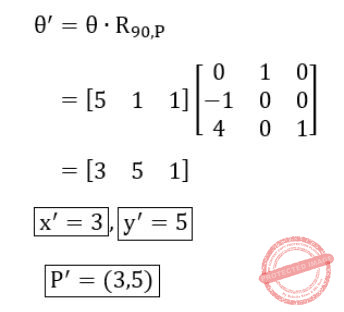Question 15
Let R be the rectangular window against which the lines are to be clipped using 2D Sutherland-Cohen line clipping algorithm. The rectangular window has a lower left-hand corner at (– 5, 1) and upper right-hand corner at (3, 7). Consider the following three lines for clipping with the given endpoint coordinates:
Line AB : A (– 6, 2) and B (–1, 8)
Line CD : C (– 1, 5) and D (4, 8)
Line EF : E (–2, 3) and F (1, 2)
Which of the following line(s) is/are candidate for clipping?
 A AB B CD C EF D AB and CD
Computer-Graphics       Sutherland-Cohen-algorithm
 Question 16
In perspective projection, if a line segment joining a point which lies in front of the viewer to a point in back of the viewer is projected to a broken line of infinite extent. This is known as _______.
 A View confusion B Vanishing point C Topological distortion D Perspective foreshortening
Computer-Graphics       Topological-Distortion
 Question 17
Let us consider that the original point is (x, y) and new transformed point is (x′, y′). Further, Shx and Shy are shearing factors in the x and y directions. If we perform the y-direction shear relative to x = xref then the transformed point is given by _______.
 A x′ = x + Shx ⋅ (y – yref) y′ = y B x′ = x y′ = y ⋅ Shx C x′ = x y′ = Shy (x – xref) + y D x′ = Shy ⋅ y y′ = y ⋅ (x – xref)
Computer-Graphics       Transformations
Question 17 Explanation:Question 18
Which of the following statement(s) is/are correct with reference to curve generation?
I. Hermite curves are generated using the concepts of interpolation.
II. Bezier curves are generated using the concepts of approximation.
III. The Bezier curve lies entirely within the convex hull of its control points.
IV. The degree of Bezier curve does not depend on the number of control points.
 A I, II and IV only B II and III only C I and II only D I, II and III only
Computer-Graphics       Bezier -Curves
 Question 19
Given the following statements: (A) To implement Abstract Data Type, a programming language require a syntactic unit to encapsulate type definition. (B) To implement ADT, a programming language requires some primitive operations that are built in the language processor. (C) C++, Ada, Java 5.0, C#2005 provide support for parameterised ADT. Which one of the following options is correct ?
 A (A), (B) and (C) are false. B (A) and (B) are true; (C) is false. C A) is true; (B) and (C) are false. D (A), (B) and (C) are true.
Programming-in-c++       Abstraction
Question 19 Explanation:
TRUE: To implement Abstract Data Type, a programming language require a syntactic unit to encapsulate type definition.

TRUE: To implement ADT, a programming language requires some primitive operations that are built in the language processor.

 Question 20
Match the following types of variables with the corresponding programming languages:A (a)-(i), (b)-(iii), (c)-(iv), (d)-(ii) B (a)-(iv), (b)-(i), (c)-(iii), (d)-(ii) C (a)-(iii), (b)-(i), (c)-(iv), (d)-(ii) D (a)-(ii), (b)-(i), (c)-(iii), (d)-(iv)
OOPS       JAVA
Question 20 Explanation:
Static variables→ Fortran 77

Stack dynamic→ Local variables in Pascal

Explicit heap dynamic→ All objects in JAVA

Implicit heap dynamic→ All variables in APL

 Question 21
Which of the following is false regarding the evaluation of computer programming languages ?
 A Application oriented features B Efficiency and Readability C Software development D Hardware maintenance cost
Operating-Systems       Basics
Question 21 Explanation:
Evaluation of computer programming languages are mainly two types.

1.External Evaluation: Rapid development, Easy maintenance, Reliability and safety, Portability, Efficiency, Low training time (learnability), Reusability and Pedagogical value

2.Internal Evaluation: Readability, Writability, Simplicity, Orthogonality, Consistency, Expressiveness and Abstraction

 Question 22
The symmetric difference of two sets S1 and S2 is defined as

S1⊖S2 = {x|x ∈ S1 or x ∈ S2, but x is not in both S1 and S2}

The nor of two languages is defined as nor (L1, L2) = {w|w |∈L1 and w |∈ L1}.

Which of the following is correct?

 A The family of regular languages is closed under symmetric difference but not closed under nor. B The family of regular languages is closed under nor but not closed under symmetric difference. C The family of regular languages are closed under both symmetric difference and nor. D The family of regular languages are not closed under both symmetric difference and nor.
Engineering-Mathematics       Set-Theory
Question 22 Explanation:
Symmetric difference, also known as the disjunctive union, of two sets is the set of elements which are in either of the sets and not in their intersection. symmetric difference of two sets S1 and S2 is defined as :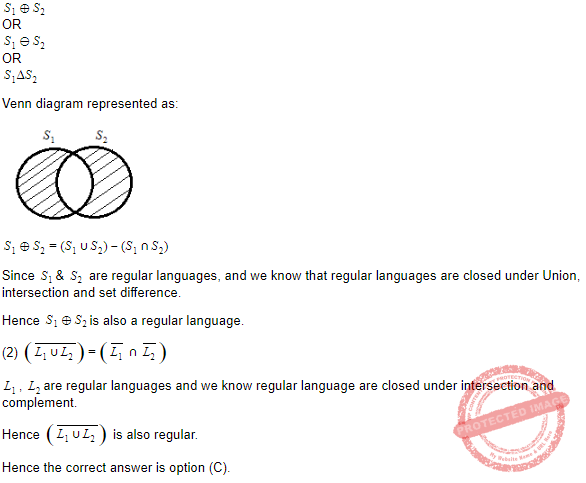Question 23
The regular expression for the complement of the language L = {anbm|n ≥ 4, m ≤ 3} is:
 A (λ + a + aa + aaa) b* + a* bbbb* + (a + b)* ba(a + b)* B (λ + a + aa + aaa) b* + a* bbbbb* + (a + b)* ab(a + b)* C (λ + a + aa + aaa) + a* bbbbb* + (a + b)* ab(a + b)* D (λ + a + aa + aaa)b* + a* bbbbb* + (a + b)* ba(a + b)*
Theory-of-Computation       Regular-Expression
Question 23 Explanation:
DFA for given language L isQuestion 24
Consider the following two languages : L1 = {0i1j| gcd (i, j) = 1} L2 is any subset of 0*. Which of the following is correct ?
 A L1 is regular and L2* is not regular B L1 is not regular and L2* is regular C Both L1 and L2* are regular languages D Both L1 and L2* are not regular languages
Theory-of-Computation       Regular-Language
Question 24 Explanation:
L1 = {0i1j| gcd (i, j) = 1}

A language could be regular if the difference between two consecutive terms is in G.P. But here L1 does not contains the strings in G.P form. Hence it is not regular language.

L2 is any subset of 0* because any subset of 0* could be {0}, {00}, {000}, {0000}, {0,00}, {0,00,000} ............... and we can have a finite automata which can accept all these subsets. Hence L2 is a regular language.

 Question 25
If link transmits 4000 frames per second and each slot has 8 bits, the transmission rate of circuit of this TDM is ______.
 A 64 Kbps B 32 Mbps C 32 Kbps D 64 Mbps
Computer-Networks       Access-Control-Methods
Question 25 Explanation:
Given data,

-- Link transmits frame per second= 4000 Frames per second

-- Each slot bits= 8 bits

-- Transmission rate of circuit of this TDM=?

Step-1: Transmission rate of circuit of this TDM= Link transmits frame per second * Each slot bits

= 4000 * 8

= 32000 bits (or 32Kbps)

 Question 26
Given the following statements:

(A) Frequency Division Multiplexing(FDM) is a technique that can be applied when the bandwidth of a link is greater than combined bandwidth of signals to be transmitted.

(B) Wavelength Division Multiplexing (WDM) is an analog multiplexing Technique to combine optical signals.

(C) WDM is a Digital Multiplexing Technique.

(D) TDM is a Digital Multiplexing Technique.

Which of the following is correct?

 A (A), (B), (C) and (D) are true. B (A), (B), (C) and (D) are false. C (A), (B) and (D) are false; (C) is true. D (A), (B) and (D) are True; (C) is false.
Computer-Networks       Access-Control-Methods
Question 26 Explanation:
In this question, if Statement-B is true then definitely Statement-C should be FALSE.

If Statement-B is FALSE then definitely Statement-C should be TRUE.

Option-A: We can ruled out based on the above two constraints.

Option-B: We can ruled out based on the above two constraints.

Option-C: Statement-B is FALSE because Wavelength Division Multiplexing (WDM) is an analog multiplexing Technique to combine optical signals.Question 27
A pure ALOHA Network transmits 200 bit frames using a shared channel with 200 Kbps bandwidth. If the system (all stations put together) produces 500 frames per second, then the throughput of the system is ______.
 A 0.384 B 0.184 C 0.286 D 0.586
Computer-Networks       Access-Control-Methods
Question 27 Explanation:
Step-1: Given data,

pure ALOHA transmits=200 bit frames

Bandwidth=200 Kbps

System produces=500 frames per second

Throughput=?

Step-2: Pure ALOHA formula S= G*e-2G

Frame transmission time=200/200 kbps=1ms

Here, G=0.5 and S=G*e-2G

=0.184 (or) 18.4%

System produces Throughput =500*0.184

=92 frames

Note: ‘e’ value should be constant. e=2.71828

 Question 28
Match the following:A (a)-(iv), (b)-(iii), (c)-(ii), (d)-(i) B (a)-(iii), (b)-(iv), (c)-(ii), (d)-(i) C (a)-(i), (b)-(iii), (c)-(ii), (iv)-(iv) D (a)-(ii), (b)-(i), (c)-(iv), (d)-(iii)
Data-Communication       PCM
Question 28 Explanation:
Line coding→ Process of converting digital data to digital signal

Block coding→ Provide redundancy to ensure synchronization and inherits error detection

Scrambling→ Provides synchronization without increasing number of bits

Pulse code modulation→ A technique to change analog signal to digital data

 Question 29
Assume that we need to download text documents at the rate of 100 pages per minute. A page is an average of 24 lines with 80 characters in each line and each character requires 8 bits. Then the required bit rate of the channel is _____.
 A 1.636 Kbps B 1.636 Mbps C 3.272 Mbps D 3.272 Kbps E None of the above
Computer-Networks       Bit-rate
Question 29 Explanation:
-- Number of Pages(P)=100 per Minute

-- Number of Lines(L)= 24

-- Number of characters(C) =80

-- One character(O) =8 bits

-- Bit rate of channel=?

Step-1: Bit rate of channel= P*L*C*O

= 100*24*80*8 bits per second

= 1536000

Step-2: Given options in Megabits per second.

= 1.536 Mbps

 Question 30
Encrypt the plain text Message “EXTRANET” using Transposition cipher technique with the following key:Using ‘Z’ as bogus character.

 A TAXERTZENZ B EXTRANETZZ C EZXZTRZANZET D EXTZRANZETZ
Computer-Networks       Network-Security
Question 30 Explanation:
Given data,

-- Plain text Message= EXTRANET

-- Bogus character=Z

-- Encrypt plain text message using Transposition cipher=?Question 31
The number of different binary trees with 6 nodes is ______.
 A 6 B 42 C 132 D 256
Data-Structures       Binary-Trees
Question 31 Explanation:
Using catalan formula to find the total number of binary trees is (2n)! / (n! * (n+1)!)

Here, n=6

= (2*6)! / (6! * (6+1)!)

= (12)! / (6! * (7)!)

= 479001600 / (720*5040)

= 132

 Question 32
Let A[1...n] be an array of n distinct numbers. If i < j and A[i] > A[j], then the pair (i, j) is called an inversion of A. What is the expected number of inversions in any permutation on n elements ?
 A θ(n) B θ(lgn) C θ(nlgn) D θ(n2)
Programming       Arrays
Question 32 Explanation:
→ Count of number of times the inner loop of insertion sort executes is actually equal to number of inversions in input permutation a1,a2,…an. Since for each value of i=1..n, j take the value 1..i−1, which means for every j < i it checks if a [ j ] > a [ i ] .

→ In any given permutation, maximum number of inversions possible is n(n-1)/2 which is O(n2). It is the case where the array is sorted in reverse order.

→ To resolve all inversions i.e., worst case time complexity of insertion sort is Θ(n2).

→ However, as per the question the number of inversions in input array is restricted to n.

→ The worst case time complexity of insertion sort reduces to Θ(n).
 Question 33
Which one of the following arrays represents a binary max-heap?
 A [26, 13, 17, 14, 11, 9, 15] B [26, 15, 14, 17, 11, 9, 13] C [26, 15, 17, 14, 11, 9, 13] D [26, 15, 13, 14, 11, 9, 17]
Data-Structures       Binary-Heap
Question 33 Explanation: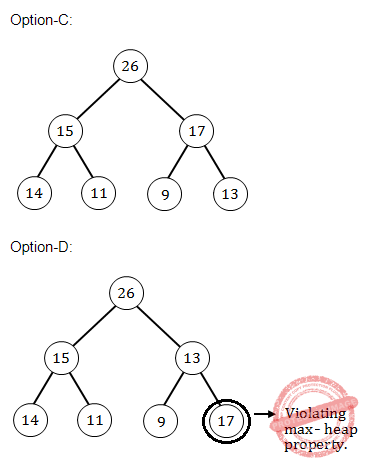Question 34
Match the following:A (a)-(i), (b)-(ii), (c)-(iv), (d)-(iii) B (a)-(i), (b)-(iv), (c)-(ii), (d)-(iii) C (a)-(iii), (b)-(ii), (c)-(iv), (d)-(i) D (a)-(iii), (b)-(iv), (c)-(ii), (d)-(i)
Software-Engineering       Software-configuration-management
Question 34 Explanation:Question 35
Suppose that we have numbers between 1 and 1,000 in a binary search tree and want to search for the number 364. Which of the following sequences could not be the sequence of nodes examined ?
 A 925, 221, 912, 245, 899, 259, 363, 364 B 3, 400, 388, 220, 267, 383, 382, 279, 364 C 926, 203, 912, 241, 913, 246, 364 D 3, 253, 402, 399, 331, 345, 398, 364
Data-Structures       Binary-Trees
Question 35 Explanation:Question 36
A triangulation of a polygon is a set of T chords that divide the polygon into disjoint triangles. Every triangulation of n-vertex convex polygon has _____ chords and divides the polygon into _____ triangles.
 A n – 2, n – 1 B n – 3, n – 2 C n – 1, n D n – 2, n – 2
Computer-Graphics       Polygon
 Question 37
Implicit return type of a class constructor is:
 A not of class type itself B class type itself C a destructor of class type D a destructor not of class type
OOPS       Constructors
Question 37 Explanation:
Implicit return type of a class constructor is class type itself.

Syntax For Constructors:

Access_Modifier No Return_Type Class(...)

Example:

public static main(String [ ]args)

Constructor rules:

1. Constructors are always used with a new.

2. Have the same name as that of Class

3. Does not have a return type

4. Can be Overloaded but not Overridden.

 Question 38
It is possible to define a class within a class termed as nested class. There are _____ types of nested classes.
 A 2 B 3 C 4 D 5
OOPS       Class-and-object
Question 38 Explanation:
Nested classes are divided into two categories:

1. Static

2. Non static.

Nested classes that are declared static are simply called static nested classes.

Non static nested classes are called inner classes.

 Question 39
Which of the following statements is correct?
 A Aggregation is a strong type of association between two classes with full ownership B Aggregation is a strong type of association between two classes with partial ownership. C Aggregation is a weak type of association between two classes with partial ownership. D Aggregation is a weak type of association between two classes with full ownership.
OOPS       Aggregation
Question 39 Explanation:
Aggregation is a special form of association. It is a relationship between two classes like association, however its a directional association, which means it is strictly a one way association. It represents a HAS-A relationship.

For example consider two classes Student class and Address class. Every student has an address so the relationship between student and address is a Has-A relationship. But if you consider its vice versa then it would not make any sense as an Address doesn’t need to have a Student necessarily.

 Question 40
Which of the following statements is correct?
(1) Every class containing abstract method must not be declared abstract.
(2) Abstract class cannot be directly initiated with ‘new’ operator.
(3) Abstract class cannot be initiated.
(4) Abstract class contains definition of implementation.
 A (1) B (2) C (2) and (3) D All are correct.
OOPS       Properties
Question 40 Explanation:
• Abstract class may consist of at least one abstract method.

• Abstract method means method without body, we can also be called as pure virtual functions.

• If a class is marked with keyword abstract then it is called an abstract class. It can NOT be instantiated by using new operator. But an abstract class can be used as the superclass reference for the subclass object.

• Abstract classes, by design, are not complete or functional. They are meant to serve as a base from which complete classes can be built, by aggregating the common members and methods that they all will need into an abstract base class, and then allowing the inheriting classes to fill out the necessary details. So abstract class can’t be instantiated

 Question 41
Which of the following statements is not correct?
 A HTML is not screen precise formatting language. B HTML does not specify a logic. C DHTML is used for developing highly interactive web pages. D HTML is a programming language.
Web-Technologies       HTML
Question 41 Explanation:
HTML is not a programming language. It is a markup language. The abbreviation of HTML is hypertext markup language.
 Question 42
When one object reference variable is assigned to another object reference variable then
 A a copy of the object is created. B a copy of the reference is created. C a copy of the reference is not created. D it is illegal to assign one object reference variable to another object reference variable.
OOPS       Class-and-object
Question 42 Explanation:
• A reference variable is an alias, that is, another name for an already existing variable. Once a reference is initialized with a variable, either the variable name or the reference name may be used to refer to the variable.

• A reference variable must be initialized at the time of declaration.

 Question 43
A server crashes on average once in 30 days, that is, the Mean Time Between Failures (MTBF) is 30 days. When this happens, it takes 12 hours to reboot it, that is, the Mean Time to Repair (MTTR) is 12 hours. The availability of server with these reliability data values is approximately :
 A 96.3% B 97.3% C 98.3% D 99.3%
Software-Engineering       Software-Reliabiliy
Question 43 Explanation:
Given data,

-- Mean Time Between Failures (MTBF)= 30 days

-- Mean Time to Repair (MTTR) =12 hours.

-- Availability=?

Step-1: First we have to convert number of days into hours

30 days= 30 * 24 = 720 hour.

Step-2: The availability of a system is

Availability = MTBF / (MTBF + MTTR)

= 720 / (720 + 12)

= 0.9836 (or) 98.36%

 Question 44
Match the software maintenance activities in List – I to its meaning in List – II.A I-(b), II-(d), III-(c), IV-(a) B I-(b), II-(c), III-(d), IV-(a) C I-(c), II-(b), III-(d), IV-(a) D I-(a), II-(d), III-(b), IV-(c)
Software-Engineering       Software-configuration-management
Question 44 Explanation:
Corrective → Concerned with fixing errors that are observed when the software is in use.

Adaptive → Concerned with the change in the software than takes place to make the software adaptable to new environment (both hardware and software).

Perfective → Concerned with the change in the software that takes place to make the software adaptable to changing user requirements

Preventive → Concerned with performing activities to reduce the software complexity thereby improving program understandability and increasing software maintainability.

 Question 45
Match each application/software design concept in List – I to its definition in List – II.A I-(b), II-(a), III-(d), IV-(c) B I-(b), II-(d), III-(a), IV-(b) C I-(d), II-(c), III-(b), IV-(a) D I-(d), II-(a), III-(c), IV-(b)
Software-Engineering       Software-design
Question 45 Explanation:
Coupling → Reliance of a code module upon other code modules

Cohesion → Focus of a code upon a single goal.

Scalable → Easy to add functionality to the software without having to redesign it.

Readable → Easy to visually inspect the design of the software and understand its purpose.

→ Cohesion is a measure of internal strength within a module, whereas coupling is a measure of inter dependency among the modules.

→ In a functional oriented design/modular software design there should be high cohesion and low coupling.

→ It requires High Fan-In and Low fan-out.

 Question 46
Software safety is quality assurance activity that focuses on hazards that
 A affect the reliability of a software component. B may cause an entire system to fail. C may result from user input errors. D prevent profitable marketing of the final product.
Software-Engineering       Software-quality
Question 46 Explanation:
Software safety: software quality assurance activity that focuses on the identification and assessment of potential hazards that may affect software negatively and cause an entire system to fail.

→ Modeling and analysis process is conducted as part of software safety.

→ Initially, hazards are identified and categorized by criticality and risk.

 Question 47
Which of the following sets represent five stages defined by Capability Maturity Model (CMM) in increasing order of maturity ?
 A Initial, Defined, Repeatable, Managed, Optimized. B Initial, Repeatable, Defined, Managed, Optimized. C Initial, Defined, Managed, Repeatable, Optimized. D Initial, Repeatable, Managed, Defined, Optimized.
Software-Engineering       CMMI
Question 47 Explanation:
CMM stands for Capability Maturity Model is a process model which specifies the process improvement approach in software development.

CMM levels:

1. Initial

2. Repeatable

3. Defined

4. Managed

5.Optimizing

 Question 48
The number of function points of a proposed system is calculated as 500. Suppose that the system is planned to be developed in Java and the LOC/FP ratio of Java is 50. Estimate the effort (E) required to complete the project using the effort formula of basic COCOMO given below : E = a(KLOC)b Assume that the values of a and b are 2.5 and 1.0 respectively.
 A 25 person months B 75 person months C 62.5 person months D 72.5 person months
Software-Engineering       COCOMO-Model
Question 48 Explanation:
Given data,

-- Functional points(FP)= 500

-- Lines of code(LOC) / FP = 50

-- Effort(E) = a(KLOC)b

-- a = 2.5 and b = 1.0

-- KLOC=?

Step-1: Find out KLOC

KLOC= ((LOC / FP)*FP)/1000

= 25

Where K=1000

Step-2: Find out Effort(E)= 2.5(25)1.0

= 62.5 person months.

 Question 49
In UNIX, processes that have finished execution but have not yet had their status collected are known as _________.
 A Sleeping processes B Stopped processes C Zombie processes D Orphan processes
Operating-Systems       UNIX-Operating-System
Question 49 Explanation:
→ An orphan process is a computer process whose parent process has finished or terminated, though it remains running itself.

→ A zombie process or defunct process is a process that has completed execution but still has an entry in the process table.

→ A process enters a Sleeping state when it needs resources that are not currently available. At that point, it either goes voluntarily into Sleep state or the kernel puts it into Sleep state. Going into Sleep state means the process immediately gives up its access to the CPU.

→ A stopped process is a stochastic process that is forced to assume the same value after a prescribed (possibly random) time.

 Question 50
In Unix operating system, when a process creates a new process using the fork () system call, which of the following state is shared between the parent process and child process?
 A Heap B Stack C Shared memory segments D Both Heap and Stack
Operating-Systems       UNIX-Operating-System
Question 50 Explanation:
A segment of memory that is shared between processes. The shared memory segments are sharing between the parent process and child process.
 Question 51
Which of the following information about the UNIX file system is not correct?
 A Super block contains the number of i-nodes, the number of disk blocks, and the start of the list of free disk blocks. B An i-node contains accounting information as well as enough information to locate all the disk blocks that holds the file’s data. C Each i-node is 256-bytes long. D All the files and directories are stored in data blocks.
Operating-Systems       UNIX-Operating-System
Question 51 Explanation:
FALSE: Each i-node is 128 bytes long.
 Question 52
Which of the following options with reference to UNIX operating system is not correct?
 A INT signal is sent by the terminal driver when one types and it is a request to terminate the current operation. B TERM is a request to terminate execution completely. The receiving process will clean up its state and exit. C QUIT is similar to TERM, except that it defaults to producing a core dump if not caught. D KILL is a blockable signal.
Operating-Systems       UNIX-Operating-System
Question 52 Explanation:
KILL

→ The kill command will kill a process using the kill signal and PID given by the user.

→ The kill command accepts either a signal number or name (signals have both a number and name that can be referenced). The name must be in all caps.

 Question 53
A multicomputer with 256 CPUs is organized as 16 × 16 grid. What is the worst case delay (in hops) that a message might have to take ?
 A 16 B 15 C 32 D 30
Computer-Organization       Memory-hierarchy
 Question 54
Suppose that the time to do a null remote procedure call (RPC) (i.e. 0 data bytes) is 1.0 msec, with an additional 1.5 msec for every 1K of data. How long does it take to read 32 K from the file server as 32 1K RPCs ?
 A 49 msec B 80 msec C 48 msec D 100 msec
Operating-Systems       RPC
Question 54 Explanation:
→ A single 32K RPC takes 1.5*32 + 1.0 = 49.0 msec

→ 32 1K RPCs take 1.5*32 + 1.0*32 = 80.0 msec

 Question 55
Let L be the language generated by regular expression 0*10* and accepted by the deterministic finite automata M. Consider the relation RM defined by M. As all states are reachable from the start state, RM has _____ equivalence classes.
 A 2 B 4 C 5 D 6
Theory-of-Computation       Finite-Automata
 Question 56
Let L = {0n1n|n ≥ 0} be a context free language. Which of the following is correct ?
 A L’ is context free and Lk is not context free for any k ≥ 1. B L’ is not context free and Lk is not context free for any k ≥ 1. C Both L’ and Lk is for any k ≥ 1 are context free. D Both L’ and Lk is for any k ≥ 1 are not context free.
Theory-of-Computation       Context-Free-Language
 Question 57
Given a Turing Machine M = ({q0, q1, q2, q3}, {a, b}, {a, b, B}, δ, B, {q3}) Where δ is a transition function defined as δ(q0, a) = (q1, a, R) δ(q1, b) = (q2, b, R) δ(q2, a) = (q2, a, R) δ(q3, b) = (q3, b, R) The language L(M) accepted by the Turing Machine is given as:
 A aa*b B abab C aba*b D aba*
Theory-of-Computation       Turing-machines
Question 57 Explanation: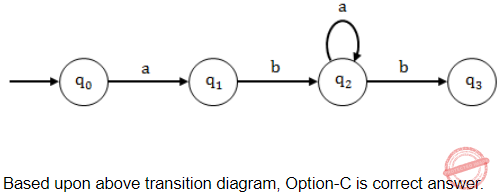Question 58
Consider a discrete memoryless channel and assume that H(x) is the amount of information per symbol at the input of the channel; H(y) is the amount of information per symbol at the output of the channel; H(x|y) is the amount of uncertainty remaining on x knowing y; and I (x; y) is the information transmission. Which of the following does not define the channel capacity of a discrete memoryless channel ?
 A max I(x; y) p(x) B max [H(y) – H(y|x)] p(x) C max [H(x) – H(x|y)] p(x) D max H(x|y) p(x)
Data-Communication       Channel-Capacity
Question 58 Explanation:
Transmission rate over a noisy channel:

→ H(X) is the amount of information per symbol at the input of the channel.

→ H(Y) is the amount of information per symbol at the output of the channel.

→ H(X|Y) is the amount of uncertainty remaining on X knowing Y.

The information transmission is given by:

I(X;Y) = H(X)−H(X|Y) bits/channel use

For an ideal channel X = Y, there is no uncertainty over X when we observe Y. So all the information is transmitted for each channel use: I(X;Y) = H(X) If the channel is too noisy, X and Y are independent. So the uncertainty over X remains the same knowing or not Y, i.e. no information passes through the channel: I(X;Y) = 0.

Capacity of a noisy channel:Question 59
Consider a source with symbols A, B, C, D with probability 1/2, 1/4, 1/8, 1/8 respectively. What is the average number of bits per symbol for the Huffman code generated from above information ?
 A 2 bits per symbol B 1.75 bits per symbol C 1.50 bits per symbol D 1.25 bits per symbol
Engineering-Mathematics       Probability
Question 59 Explanation: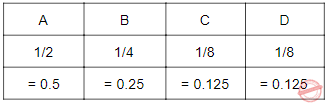Question 60
Which of the following is used for the boundary representation of an image object?
 A Quad Tree B Projections C Run length coding D Chain codes
Image-Processing       Chain-Codes
Question 60 Explanation:
→ Chain codes is used for the boundary representation of an image object.

→ A chain code is a lossless compression algorithm for monochrome images. The basic principle of chain codes is to separately encode each connected component, or "blob", in the image.

→ For each such region, a point on the boundary is selected and its coordinates are transmitted. The encoder then moves along the boundary of the region and, at each step, transmits a symbol representing the direction of this movement.

 Question 61
The region of feasible solution of a linear programming problem has a _____ property in geometry, provided the feasible solution of the problem exists.
 A concavity B convexity C quadratic D polyhedron
LPP       Convexity
Question 61 Explanation:
→ The region of feasible solution of an LP problem has a convexity property in geometry, provided the feasible solution of the problem exists.

→ The basic feasible solutions for a standard form LP are vertices for the convex set of feasible solution for the LP.

→ If an LP has a ﬁnite optimal objective function value, then the optimal value occurs at at least one basic feasible solution.

 Question 62
Consider the following statements:
(a) Revised simplex method requires lesser computations than the simplex method.
(b) Revised simplex method automatically generates the inverse of the current basis matrix.
(c) Less number of entries are needed in each table of revised simplex method than usual simplex method.
Which of these statements are correct?
 A (a) and (b) only B (a) and (c) only C (b) and (c) only D (a), (b) and (c)
LPP       Simplex
 Question 63
The following transportation problem: isA infeasible solution B optimum solution C non-optimum solution D unbounded solution
LPP       Transportation-Problem
 Question 64
Let R and S be two fuzzy relations defined as:Then, the resulting relation, T, which relates elements of universe X to elements of universe Z using max-min composition is given by

 ABCDArtificial-intelligence       Fuzzy-sets
Question 64 Explanation: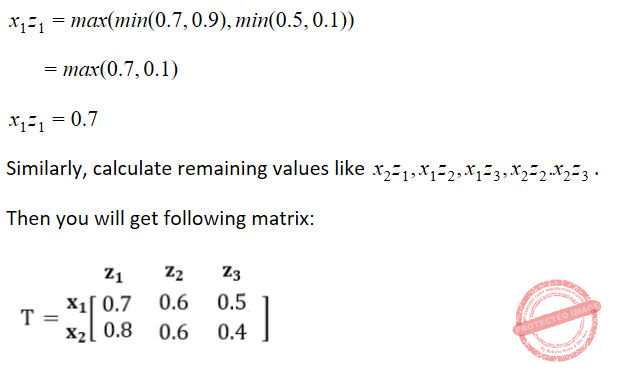Question 65
Compute the value of adding the following two fuzzy integers :
A = {(0.3, 1), (0.6, 2), (1, 3), (0.7, 4), (0.2, 5)}
B = {(0.5, 11), (1, 12), (0.5, 13)}
Where fuzzy addition is defined as μA+B (z)= maxx + y = z(min (μA(x), μB(x)))
Then, f (A + B) is equal to
 A {(0.5, 12), (0.6, 13), (1, 14), (0.7, 15), (0.7, 16), (1, 17), (1, 18)} B {(0.5, 12), (0.6, 13), (1, 14), (1, 15), (1, 16), (1, 17), (1, 18)} C {(0.3, 12), (0.5, 13), (0.5, 14), (1, 15), (0.7, 16), (0.5, 17), (0.2, 18)} D {(0.3, 12), (0.5, 13), (0.6, 14), (1, 15), (0.7, 16), (0.5, 17), (0.2, 18)}
Artificial-intelligence       Fuzzy-sets
Question 65 Explanation:Question 66
A perceptron has input weights W1 = – 3.9 and W2 = 1.1 with threshold value T = 0.3. What output does it give for the input x1 = 1.3 and x2 = 2.2 ?
 A – 2.65 B – 2.30 C 0 D 1
Artificial-intelligence       Neural-Networks
Question 66 Explanation:Question 67
What is the function of following UNIX command ?
WC – l <a  >b&
 A It runs the word count program to count the number of lines in its input, a, writing the result to b, as a foreground process. B It runs the word count program to count the number of lines in its input, a, writing the result to b, but does it in the background. C It counts the errors during the execution of a process, a, and puts the result in process b. D It copies the ‘l’ numbers of lines of program from file, a, and stores in file b.
Operating-Systems       UNIX-Operating-System
Question 67 Explanation:
wc [options] filenames
WC → Word count
Options:
wc -l : Prints the number of lines in a file.
wc -w : prints the number of words in a file.
wc -c : Displays the count of bytes in a file.
wc -m : prints the count of characters from a file.
wc -L : prints only the length of the longest line in a file.
WC – l < a >b& → It runs the word count program to count the number of lines in its input, a, writing the result to b, but does it in the background.
 Question 68
Which of the following statements is not correct with reference to cron daemon in UNIX O.S. ?
 A The cron daemon is the standard tool for running commands on a predetermined schedule. B It starts when the system boots and runs as long as the system is up. C Cron reads configuration files that contain list of command lines and the times at which they invoked. D Crontab for individual users are not stored.
Operating-Systems       UNIX-Operating-System
Question 68 Explanation:
→ The cron daemon is a long-running process that executes commands at specific dates and times.

→ The crontab command creates a crontab file containing commands and instructions for the cron daemon to execute.

→ The cron daemon is the standard tool for running commands on a predetermined schedule.

→ It starts when the system boots and runs as long as the system is up.

→ Cron reads configuration files that contain list of command lines and the times at which they invoked.

 Question 69
In Unix, files can be protected by assigning each one a 9-bit mode called rights bits. Now, consider the following two statements:

I. A mode of 641 (octal) means that the owner can read and write the file, other members of the owner’s group can read it, and users can execute only.

II. A mode of 100 (octal) allows the owner to execute the file, but prohibits all other access.

Which of the following options is correct with reference to the above statements ?
 A Only I is correct. B Only II is correct. C Both I and II are correct. D Both I and II are incorrect.
Operating-Systems       Linux-Commands
Question 69 Explanation:
To change file permissions we are using chmod and chown. To display all permissions using ls -l command.Question 70
Consider the statement,
“Either – 2 ≤ x ≤ – 1 or 1 ≤ x ≤ 2”.
The negation of this statement is
 A x < – 2 or 2 < x or – 1 < x < 1 B x < – 2 or 2 < x C – 1 < x < 1 D x ≤ – 2 or 2 < x or – 1 < x < 1
Programming       Operator
 Question 71
Which of the following is characteristic of an MIS?
 A Provides guidance in identifying problems, finding and evaluating alternative solutions, and selecting or comparing alternatives. B Draws on diverse yet predictable data resources to aggregate and summarize data. C High volume, data capture focus. D Has as its goal the efficiency of data movement and processing and interfacing different TPS.
Database-Management-System       MIS
Question 71 Explanation:
→ A management information system (MIS) is an information system used for decision-making, and for the coordination, control, analysis, and visualization of information in an organization.

→ MIS Draws on diverse yet predictable data resources to aggregate and summarize data.

 Question 72
How does randomized hill-climbing choose the next move each time?
 A It generates a random move from the moveset, and accepts this move. B It generates a random move from the whole state space, and accepts this move. C It generates a random move from the moveset, and accepts this move only if this move improves the evaluation function. D It generates a random move from the whole state space, and accepts this move only if this move improves the evaluation function.
Artificial-intelligence       Hill-Climbing-Algorithm
Question 72 Explanation:
Randomized hill-climbing generates a random move from the moveset, and accepts this move only if this move improves the evaluation function.
 Question 73
Consider the following game tree in which root is a maximizing node and children are visited left to right. What nodes will be pruned by the alpha-beta pruning ?A I B HI C CHI D GHI
Artificial-intelligence       Alpha-Beta pruning
 Question 74
Consider a 3-puzzle where, like in the usual 8-puzzle game, a tile can only move to an adjacent empty space. Given the initial statewhich of the following state cannot be reached?
 ABC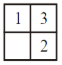DArtificial-intelligence       3-Puzzle-Game
Question 74 Explanation:
This problem, we can solve it by presence of mind.Question 75
A software program that infers and manipulates existing knowledge in order to generate new knowledge is known as
 A Data dictionary B Reference mechanism C Inference engine D Control strategy
Artificial-intelligence       Inference-Engine
Question 75 Explanation:
→ A software program that infers and manipulates existing knowledge in order to generate new knowledge is known as inference engine.

→ Inference engines work primarily in one of two modes either special rule or facts: forward chaining and backward chaining.

→ Forward chaining starts with the known facts and asserts new facts.

→ Backward chaining starts with goals, and works backward to determine what facts must be asserted so that the goals can be achieved

There are 75 questions to complete.